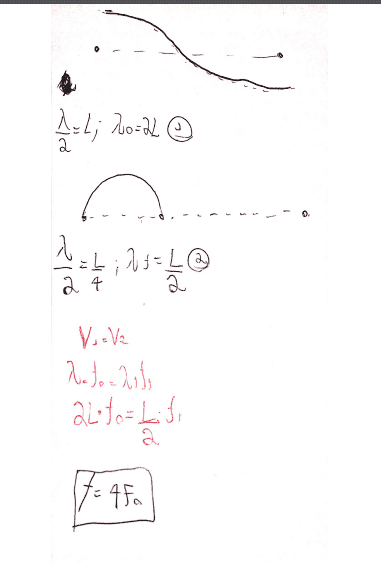# Fundamental frequencies in a violin string.

LCSphysicist
Homework Statement:
A violin string on a violin is of length L and can be considered to be
fastened at both ends. The fundamental of the open string has a frequency
fo. The violinist bows the string at a distance L/4 from one end and touches
the string lightly at the midpoint.
"Under this condition, what is the lowest frequency he can excite?"
Relevant Equations:
All below
Why is this wrong?:That is, why is not f = 4fo?
Oh. And my figure 1 can be wrong, because maybe i got confused if the string was open or closed in the boundaries, anyway this change nothing the relations (1)

Homework Helper
Homework Statement:: A violin string on a violin is of length L and can be considered to be
fastened at both ends.
The fundamental of the open string has a frequency
fo. The violinist bows the string at a distance L/4 from one end and touches
the string lightly at the midpoint.

"Under this condition, what is the lowest frequency he can excite?"
Relevant Equations:: All below

Why is this wrong?:
View attachment 266711

That is, why is not f = 4fo?
Oh. And my figure 1 can be wrong, because maybe i got confused if the string was open or closed in the boundaries, anyway this change nothing the relations (1)
The string is fastened at both ends.
The violinist bows the string at a distance L/4 from one end and touches
the string lightly at the midpoint.

Where are the nodes and antinodes of the string? What is the wavelength then?

•LCSphysicist
LCSphysicist
The string is fastened at both ends.
The violinist bows the string at a distance L/4 from one end and touches
the string lightly at the midpoint.

Where are the nodes and antinodes of the string? What is the wavelength then?
Maybe...
The antinodes is where the violinist touch, and the nodes are just the points which he is holding and the another normal point which was stuck from the beginning.
L/4 = λ/4
L = λ.
This solve the problems.

Homework Helper
Maybe...
The antinodes is where the violinist touch, and the nodes are just the points which he is holding and the another normal point which was stuck from the beginning.
L/4 = λ/4
L = λ.
This solve the problems.
yes, so the lowest frequency heard is?